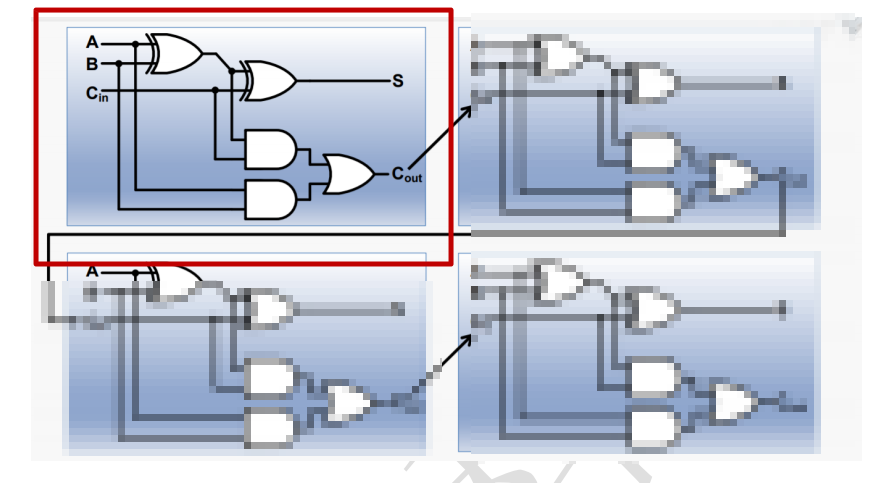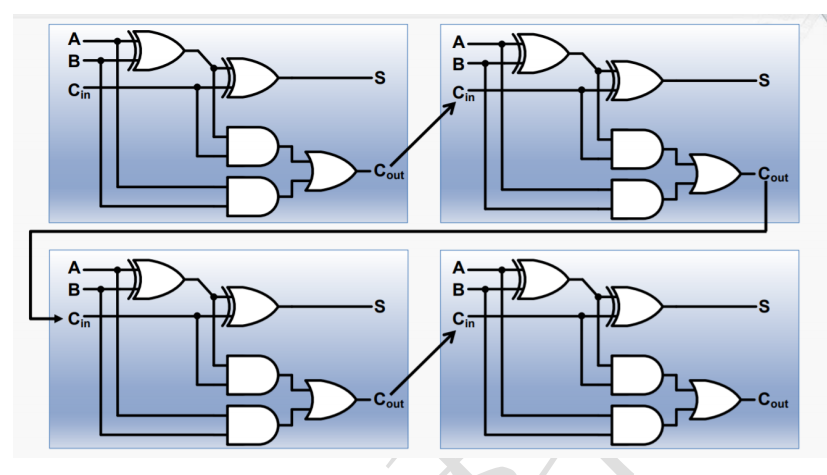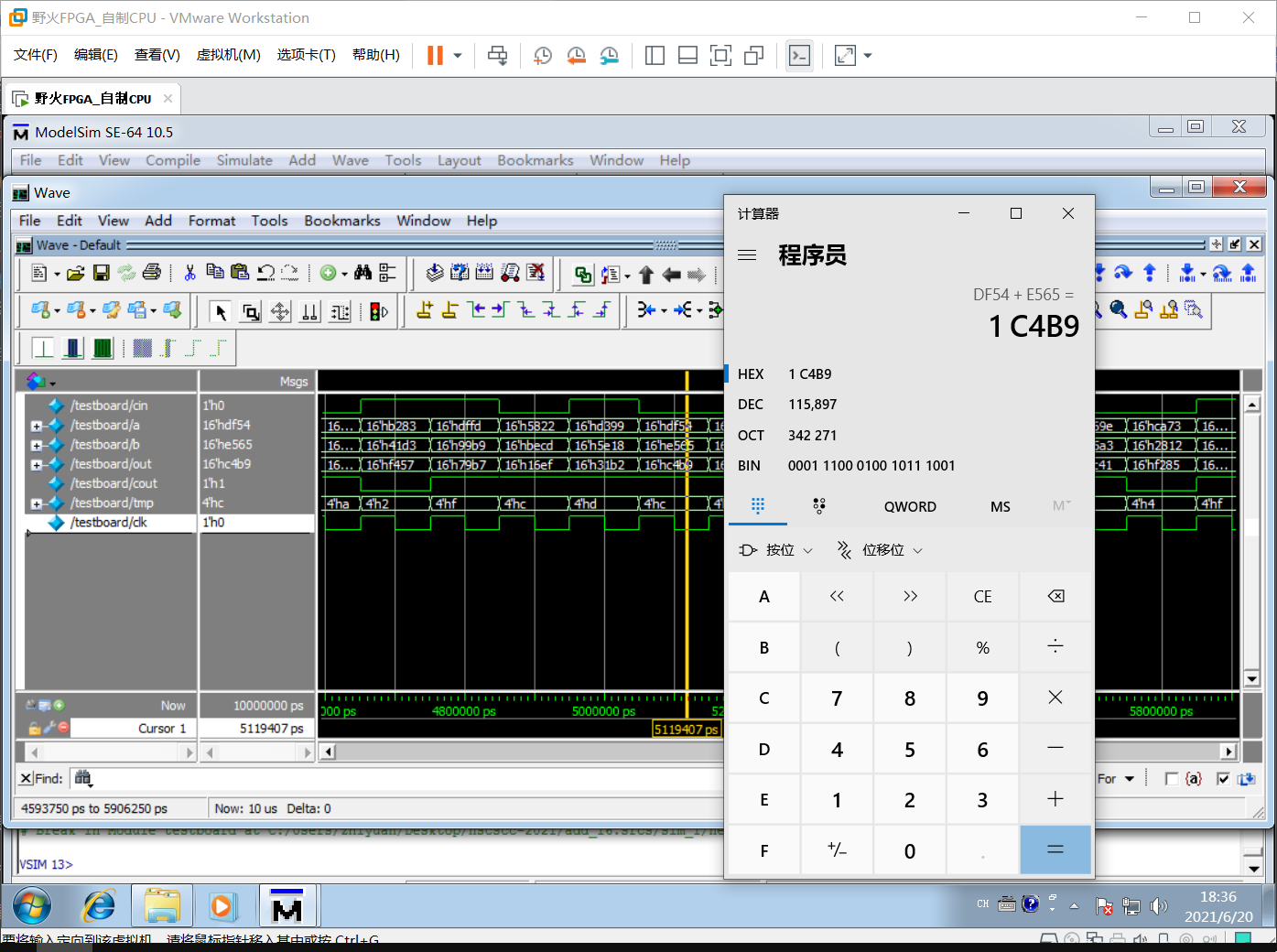# 实现最底层的 4 位加法器（门电路构成）S = (A ^ B) ^ Cin ;

Cout = ((A ^ B) . Cin) + (A . B) ;

Ps：“.”是与、“+”是或。

   // 根据加法器实验结构图写出下面的基本逻辑门
assign out = a^b^cin;                       // 电路图中的S
assign temp = (a&b)|((a^b)&cin);      // 电路图中的Coutmodule add_4(a, b, cin, out, cout);
input [3:0] a;
input [3:0] b;
input cin;          // 前一位进位
output [3:0] out;
output cout;        // 进位
wire [3:0] temp;

// 根据加法器实验结构图写出下面的基本逻辑门
assign out = a^b^cin;                       // 电路图中的S
assign temp = (a&b)|((a^b)&cin);      // 电路图中的Cout

assign out = a^b^temp;
assign temp = (a&b)|((a^b)&temp);

assign out = a^b^temp;
assign temp = (a&b)|((a^b)&temp);

assign out = a^b^temp;
assign temp = (a&b)|((a^b)&temp);

assign cout = temp;

endmodule

# 由 4 个 4 位加法器串联，构成中层 16 位加法器

timescale 1ns / 1ps
//////////////////////////////////////////////////////////////////////////////////
// Company:
// Engineer:
//
// Create Date: 2017/10/17 09:52:05
// Design Name:
// Project Name:
// Target Devices:
// Tool Versions:
// Description:
//
// Dependencies:
//
// Revision:
// Revision 0.01 - File Created
//
//////////////////////////////////////////////////////////////////////////////////

module add_4(a, b, cin, out, cout);
input [3:0] a;
input [3:0] b;
input cin;          // 前一位进位
output [3:0] out;
output cout;        // 进位
wire [3:0] temp;

// 根据加法器实验结构图写出下面的基本逻辑门
assign out = a^b^cin;                       // 电路图中的S
assign temp = (a&b)|((a^b)&cin);      // 电路图中的Cout

assign out = a^b^temp;
assign temp = (a&b)|((a^b)&temp);

assign out = a^b^temp;
assign temp = (a&b)|((a^b)&temp);

assign out = a^b^temp;
assign temp = (a&b)|((a^b)&temp);

assign cout = temp;

endmodule

module add_16(a, b, cin, out, cout, tmp);
input [15:0] a;
input [15:0] b;
input cin;
output [15:0] out;
output cout;
output [3:0] tmp;
wire [3:0] temp1;

.a(a[3:0]),
.b(b[3:0]),
.cin(cin),
.out(out[3:0]),
.cout(temp1)
);
.a(a[7:4]),
.b(b[7:4]),
.cin(temp1),
.out(out[7:4]),
.cout(temp1)
);

.a(a[11:8]),
.b(b[11:8]),
.cin(temp1),
.out(out[11:8]),
.cout(temp1)
);
.a(a[15:12]),
.b(b[15:12]),
.cin(temp1),
.out(out[15:12]),
.cout(temp1)
);

assign cout = temp1;
assign tmp = temp1;

endmodule

`# 由 4 个 16 位加法器串联，构成上层 64 位加法器

https://www.runoob.com/w3cnote/verilog-expression.html

https://images.app.goo.gl/UPpoGDEoepcnPZF86